# SQL JoinsThe BI School aims to be a guiding light for people trying to learn the SQL language

In this section of the website we provide definitions of some common SQL commands with examples and descriptions. Please browse these pages as much as you like to set you on your way with SQL.

If you're still getting stuck, please consider our training programs. Teaching SQL is our speciality.

SQL Library

Joins

other stuff...

SQL Joins

SQL joins can be a bit tricky, so let me explain...

A Join is a means of selecting data from two (or more) tables at once. Not just selecting either, you can update and delete using joins too.

Consider these two tables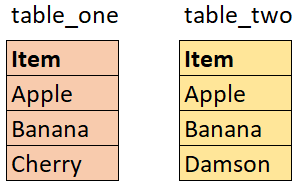First point is this. In these examples table_one will always come immediately after the FROM and before the JOIN, so we will regard that as the left table. After the JOIN comes table_two so we should think of that as the right table as it is on the right of the other table.

Notice how items 1 and 2 (Apple and Banana) are the same on both tables but the third rows are different...

The basic two table query with a simple join condition looks like this:

SELECT *

FROM [table_one]

[______] JOIN [table_Two] ON [table_one].Item = [table_two].Item

Where [______] is the type of join. As you will see, there are six types of join and we'll discuss them all here.

In the above query, I have used the word "JOIN" to join the tables

Using "JOIN" like this is the same as using "INNER JOIN".

"INNER JOIN" is the first of SIX join types and it means that when this query is run, the query will show only the rows that appear in both tables.

So in the output (the result of the query), we would see something like this: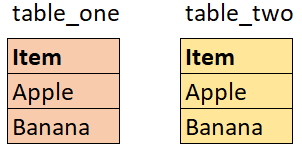We don't see Cherry from table_one because it doesn't appear in table_two, and we don't see Damson because it doesn't appear in table_one

The next type of join is the LEFT JOIN (sometimes referred to as a LEFT OUTER JOIN).

We'll use the same two tables.

The definition of a LEFT JOIN is this: The query will show ALL rows from the left table, and only the rows from the right table where there is matching data in the left table.

SELECT *

FROM [table_one]

LEFT JOIN [table_Two] ON [table_one].Item = [table_two].Item

The result from this query is as follows: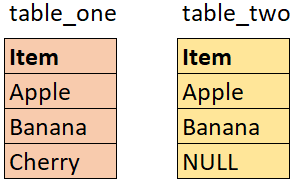We see all the rows from table_one, and where there are matches in table_two we see those too (Apple and Banana). Where there is no match, a NULL is returned. A NULL indicates the absence of any data.

The LEFT JOIN can generally be regarded as the 'safe option' because you know you aren't going to miss any data records from the left table.

The opposite of the LEFT JOIN is, you've guessed it, the RIGHT JOIN.

A RIGHT JOIN, as the name suggests, does the opposite of the left join.

SELECT *

FROM [table_one]

RIGHT JOIN [table_Two] ON [table_one].Item = [table_two].Item

The result of this query would be the following: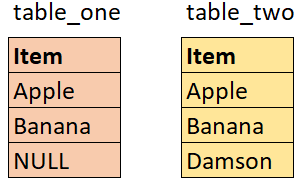Here we have all the rows from the right table (table_two) and only the rows from the left table (table_one) where there are matching rows.

The BI School would advise NEVER to use a RIGHT JOIN and the reasons for that are as follows:

Everything a RIGHT JOIN can do, a LEFT JOIN can do better.

It does not make logical sense to start with the left table, but then show all the records from the right table. It would make much more sense to simply reverse the tables and use a LEFT JOIN.

In twenty years of writing SQL, I have never used a RIGHT JOIN. There's just no need for it. The only time I ever see a RIGHT JOIN in use, is when the query has been created via a drag and drop wizard which builds the SQL in the background.

If you ever see a RIGHT JOIN in use, my sincere advice would be to spend some time switching the tables about so that you can use a LEFT JOIN instead.

Probably 95% of the joins you will use in your work with SQL will be either INNER JOINS or LEFT JOINS.

Next up, we have the FULL JOIN.

A FULL JOIN will return all the rows from both tables,. Where there are no matching rows, NULLs will be returned.

SELECT *

FROM [table_one]

FULL JOIN [table_Two] ON [table_one].Item = [table_two].Item

The result of this query would be the following: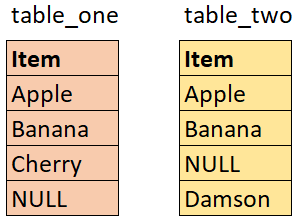As you can imagine. It makes no difference to the data output which way round the tables are listed in a FULL JOIN.

You could have FROM table_two FULL JOIN table_one and you'd get the same result, although perhaps ordered differently.

A FULL JOIN can be very useful when comparing two datasets. You can see which values appear in one data set but not the other and vice versa.

The fifth type of join is a CROSS JOIN

A CROSS JOIN will return a cartesian product of the two tables, that is it will show every matched pair. So the number of records returned will equal [number of records in the left table] * [number of records in the right table].

A cross join is fundamentally different in that it does not require a join condition. The query looks like this:

SELECT *

FROM [table_one]

CROSS JOIN [table_Two]

And this will return: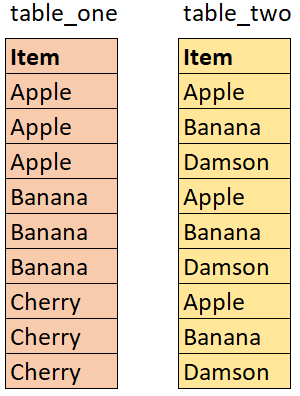So for every Apple in table_one, you've got an Apple, a Banana and a Damson in table_two. Then for every Banana in table_one, you've again got an Apple, a Banana and a Damson in table_two and so forth.

Three rows in table one, three in table_two so your output has nine rows.

You need to be careful when you use a cross join because if you have 1000 rows in one table and 1000 in another. CROSS JOIN those together and you have a million rows.

CROSS JOIN is rarely used but can be very useful, when employed correctly.

The final type of join is a SELF JOIN, and this is where a table is joined to itself!

We can't use table_one and table_two in this example, so we'll introduce a new table and call it table_three.

A self join is where a field in a table can be joined to another field, but in the same table.

The table below contains a person_id and a manager_id. The manager_id refers to the person_id in the same table. For example Adam, Barbara and Chris all have the same manager and that person has the manager_id of 4.

That manager_id field relates back to the person_id, meaning that David is the person who the other three all report to.

David does not have a manager_id, because he is the highest person in this hierarchy.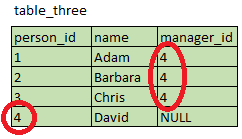The query to extract the person and their manager is as follows

SELECT emp.person_id

,emp.name as employee_name

,man.name as manager_name

FROM table_three emp

LEFT JOIN table_three man on emp.manager_id = man.person_id

You'll notice that I've used a LEFT JOIN. There is no join type called a self join. Self join is simply the name given to the technique of joining a table to itself in this manner. It would normally require an INNER JOIN or a LEFT JOIN.

The result for the above query would be as follows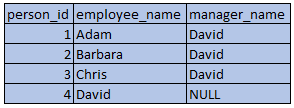So that's joins. If you want to dig deeper into SQL, please feel free to book a call to talk to Nick so we can discuss your best way forward.

Thanks,

Nick

The BI School